@l1ll5 2020-02-11T04:00:12.000000Z 字数 12657 阅读 1728

# 基础数据结构杂谈

—— by 东北师范大学附属中学 l1ll5

## 写在前面的话## Q&A

Q：你是哪个菜？我怎么没听说过你。
A：我的确是个菜...
所以没法掏出一堆很屌的个人成就来让你认识我...
Q：如果我事后发现了文章的错误想骂你该怎么办？
A：你可以加 QQ：2817629709 去骂我，或者在我的博客下留言。
Q：如果我现在就发现了文章的错误想骂你该怎么办？
A：那你就赶快拍桌子大喊 異議あり骂我吧~
Q：你讲的东西太水我想睡觉怎么办？
A：请睡吧...
Q：你讲的东西太神我听不懂怎么办？
A：那你一定是在假...

## 数据结构

data structure

## 单调栈

### 例 $1$

（可能和原题意不完全一致，在此不考虑等于的边界情况。）

1. 该旧元素没被更新过。
2. 该旧元素小于新进元素。

BZOJ 1660 乱发节

## 树状数组

( Binary Indexed Tree )

1. $add$ $x$ $y$：令 $a_x=a_x+y$
2. $ask$ $l$ $r$：查询 $sum_{i=l}^{r} a_i$

$n,m \leq 10^6$

$c_1=a_1$
$c_2=a_1+a_2$
$c_3=a_3$
$c_4=a_1+a_2+a_3+a_4$
$c_5=a_5$
$c_6=a_5+a_6$
$c_7=a_7$
$c_8=a_1+a_2+...+a_8$
$...$
$c_{16}=a_1+a_2+...+a_{16}$

$c_i$维护了 $(x-lowbit_x,x]$这个区间的信息。

int ask(int x){    int ret=0;    for(;x;x-=lowbit(x))    ret+=c[x];    return ret;}

$c$ 数组拆分了区间，每次将这些区间的贡献累加起来。
$\Delta x=x-lowbit(x)$ ，则首先累计 $(\Delta x,x]$ 的答案，再考虑 $(\Delta x-lowbit_{\Delta x},\Delta x]$的答案...以此类推。

void add(int x,int v){    for(;x<=n;x+=lowbit(x))    c[x]+=v;}

$k-lowbit_k < x \leq k$
$k$$x$ 的二进制组成一定是形如这样的：
$k:X10000$
$x:X01000$

$p：Y1Z$• 方便好写
• 常数很小，实际运行速度飞快
• 不能解决大多数问题

### 一个比较常见的小技巧：树状数组维护区间加和区间求和。

$sum_i = \sum_{j=1}^{i} a_j + det_1 \times i+det_2\times (i-1) + ... +det_i\times 1$
$sum_i = \sum_{j=1}^{i} a_j + \sum_{j=1}^{i}det_j\times (i-j+1)$
$sum_i = \sum_{j=1}^{i} a_j + (i+1) \sum_{j=1}^{i}det_j - \sum_{j=1}^{i}det_j\times j$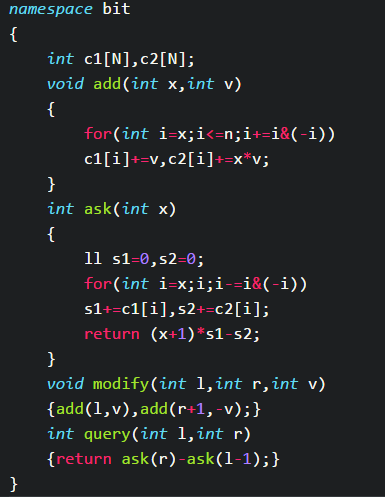### 例 $1$

$n,m \leq 5 \times 10^5 , 0 \leq x_i,y_i \leq 10^9$BZOJ 1935 [Shoi2007]Tree 园丁的烦恼

### 例 $2$

$n,m,a_i \leq 10^6$BZOJ 2743 [HEOI2012] 采花

## 线段树

### 普通线段树：

1. $add$ $x$ $y$：令 $a_x=a_x+y$
2. $ask$ $l$ $r$：查询 $sum_{i=l}^{r} a_i$

$n,m \leq 2\times 10^5$### 文艺线段树：

1. $add$ $l$ $r$ $y$：令对于所有 $x \in [l,r]$$a_x=a_x+y$
2. $ask$ $l$ $r$：查询 $sum_{i=l}^{r} a_i$

$n,m \leq 2\times 10^5$

1. 修改可下传性，即可快速计算修改操作对子区间的影响。
2. 查询可上传性，即可快速用子区间的信息修改母区间的信息。

### 例 $1$

$n \leq 5 \times 10^5 , m \leq 10^5$1. 左全选，右选左起
2. 右全选，左选右起
3. 左右全选

BZOJ 1756 Vijos1083 小白逛公园

### 例 $2$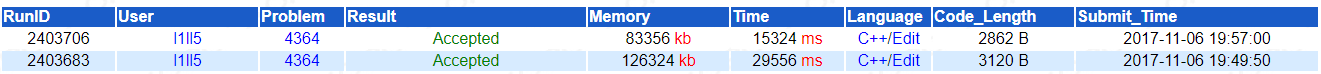BZOJ 4364 [IOI2014]wall砖墙

### 例 $3$int len[N<<2],L[N<<2],R[N<<2],cov[N<<2];//最多连续01 左右连续01 覆盖int sum[N<<2],rev[N<<2];//求和 取反void pushup(int x,int l,int r){    int ls=x<<1,rs=x<<1|1;    int mid=(l+r)>>1;    sum[x]=sum[ls]+sum[rs];    len[x]=Mx(len[ls],len[rs]);    len[x]=Mx(len[x],R[ls]+L[rs]);    len[x]=Mx(len[ls],len[rs]);    len[x]=Mx(len[x],R[ls]+L[rs]);    L[x]=L[ls]+(L[ls]==mid-l+1)*L[rs];    L[x]=L[ls]+(L[ls]==mid-l+1)*L[rs];    R[x]=R[rs]+(R[rs]==r-mid)*R[ls];    R[x]=R[rs]+(R[rs]==r-mid)*R[ls];}void pushdown(int x,int l,int r){    int ls=x<<1,rs=x<<1|1;    int mid=(l+r)>>1;    if(cov[x]!=-1)    {        if(cov[x]==0)        {            rev[ls]=rev[rs]=0;            sum[ls]=sum[rs]=0;            cov[ls]=cov[rs]=0;            len[ls]=len[rs]=L[ls]=L[rs]=R[ls]=R[rs]=0;            len[ls]=R[ls]=L[ls]=mid-l+1;            len[rs]=R[rs]=L[rs]=r-mid;        }        else        {            rev[ls]=rev[rs]=0;            len[ls]=L[ls]=R[ls]=sum[ls]=mid-l+1;            len[rs]=L[rs]=R[rs]=sum[rs]=r-mid;            cov[ls]=cov[rs]=1;            len[ls]=len[rs]=L[ls]=L[rs]=R[ls]=R[rs]=0;        }    }    if(rev[x])    {        rev[ls]^=1,rev[rs]^=1;        sum[ls]=mid-l+1-sum[ls];        sum[rs]=r-mid-sum[rs];        swap(len[ls],len[ls]);        swap(len[rs],len[rs]);        swap(L[ls],L[ls]);        swap(L[rs],L[rs]);        swap(R[ls],R[ls]);        swap(R[rs],R[rs]);    }    rev[x]=0;cov[x]=-1;}

BZOJ 1858 [Scoi2010] 序列操作

### 例 $4$

void update(int &x,int l,int r,int val){    if(!x)x=++tot;    if(l>=L&&r<=R)    {        v[x]=max(v[x],val);        return ;    }    int mid=(l+r)>>1;    if(L<=mid)    update(ch[x],l,mid,val);    if(R>mid)    update(ch[x],mid+1,r,val);}

BZOJ 4636 蒟蒻的数列

### 例 $5$

$(a,b)$,$(c,d)$表示，对于任意两个点 $x,y$，如果 $a \leq x \leq b$,$c \leq y \leq d$，那么在$x,y$之间建造一条边，边权为 $1$

$n,m \leq 5 \times 10^5$（对于给出的加边操作 由于其为无向边，在进行下操作后进行一次反操作。）

BZOJ 1636/1699 Balanced Lineup 排队
BZOJ 5039 [Jsoi2014] 序列维护
BZOJ 2770 YY 的 Treap
BZOJ 4631 踩气球
BZOJ 3939 [Usaco2015 Feb]Cow Hopscotch
BZOJ 3038 上帝造题的七分钟 2

## 主席树void update(int &x,int y,int l,int r,int pos){    x=++tot;    ch[x]=ch[y],ch[x]=ch[y],sum[x]=sum[y]+1;    if(l==r)return ;    int mid=(l+r)>>1;    if(pos<=mid) update(ch[x],ch[y],l,mid,pos);    else update(ch[x],ch[y],mid+1,r,pos);}

### 二维数点问题

$n \leq 5 \times 10^5 ， 1 \leq x_i,y_i \leq n$namespace seg{    int ch[N*40],sum[N*40],tot;    void update(int &x,int y,int l,int r,int pos)    {        x=++tot;        ch[x]=ch[y],ch[x]=ch[y],sum[x]=sum[y]+1;        if(l==r)return ;        int mid=(l+r)>>1;        if(pos<=mid) update(ch[x],ch[y],l,mid,pos);        else update(ch[x],ch[y],mid+1,r,pos);    }    int ask(int x,int l,int r,int L,int R)    {        if(l>=L&&r<=R)return sum[x];        int mid=(l+r)>>1,ret=0;        if(L<=mid) ret+=ask(ch[x],l,mid,L,R);        if(R>mid)  ret+=ask(ch[x],mid+1,r,L,R);        return ret;    }}
int now=1;for(int i=1;i<=n;i++){    root[i]=root[i-1];    while(now<=cnt&&b[now].y==i)    seg::update(root[i],root[i],1,n,b[now].x),now++;}//每次加入当前行的点

### 例 $1$POJ 2104 K-th Number

### 例 $2$

$n,m \leq 5\times 10^5$BZOJ 3524 [Poi2014]Couriers

### 例 $3$

$n,m \leq 5\times 10^5$BZOJ 2588 Spoj 10628. Count on a tree

### 例 $4$

• $1$ $a$ $b$ 合并 $a$,$b$ 所在集合
• $2$ $k$ 回到第 $k$ 次操作之后的状态 (查询算作操作)
• $3$ $a$ $b$ 询问 $a$,$b$ 是否属于同一集合，是则输出 $1$， 否则输出 $0$

$n,m \leq 5\times 10^5$BZOJ [3673/3674] [可持久化并查集 by zky]/[可持久化并查集加强版]

### 例 $5$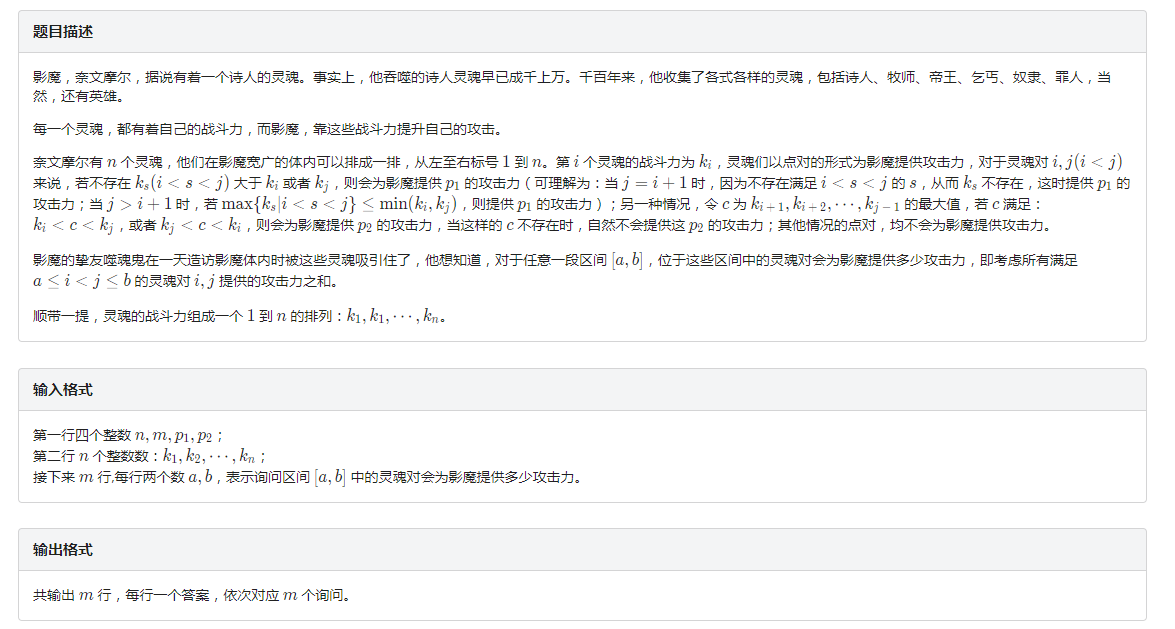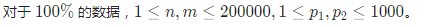$pre_i$$nxt_i$ 分别表示 $i$ 前后第一个比它大的数的位置。

BZOJ 4826 [Hnoi2017] 影魔

BZOJ 3123 [Sdoi2013] 森林
BZOJ 4771 七彩树

## 写在后面的话• 私有
• 公开
• 删除